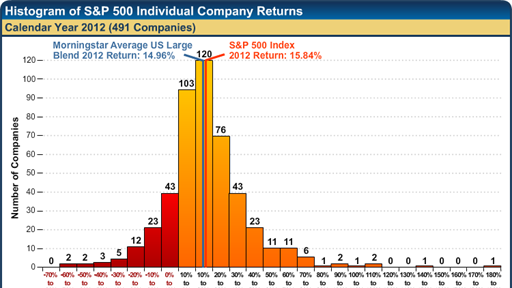# Earnings Growth and Stock Returns

At first glance, equation (2) appears to refute the assertion that expected return and earnings growth are unrelated. It seems to indicate that, given the dividend yield, the higher the growth rate, the higher the expected rate of return. But this is not a correct interpretation of equation (2). What equation (2) shows is the decomposition of the market-determined expected total rate of return into the portion that is dividend yieldand the portion that is capital appreciation (g).

If investors are indifferent between a dollar of dividends and a dollar of capital gains, financial markets will determine share prices that equate expected total rates of return for identical risks. The breakdown of the total return into its dividend and capital gains components is irrelevant.5 As seen in table 1, the earnings growth rate determines the rate of capital appreciation. Company A has a dividend yield of 10 percent and 5 percent capital gains. Company B has a dividend yield of 5 percent and 10 percent capital gains. B, with higher earnings growth, has more capital appreciation and less dividend yield than A, but the expected total returns of A and B must both be 15 percent because their risks are identical.

On March 7, 2000, the price of Procter & Gamble shares fell 31 percent on a surprise announcement that earnings would be much lower than previously anticipated. Referring to equation (1), the DDM indicates that share prices should react to earnings revisions. For example, given an expected return of 15 percent, the price of B is \$20 initially when next year's expected EPS is \$5, the payout ratio is 20 percent, and the expected earnings growth rate is 10 percent. If new information causes a reduction in the expected earnings growth rate to 7.5 percent (while next year's expected EPS and the payout ratio remain constant), the price of B must drop by 33 percent to \$13.33 to maintain the equilibrium 15 percent expected rate of return. (B's price also will drop to \$13.33 if next year's expected EPS is revised downward to \$3.33 while the expected earnings growth rate remains 10 percent and the payout ratio remains 20 percent.)6

Conclusions

• Earnings growth does not affect expected total rates of return. Expected returns are determined by risk, and the greater the risk, the higher the expected rate of return.
• For a given expected total rate of return, the earnings growth rate determines the breakdown of total returns into dividend yield and capital gains. All else the same, a higher earnings growth rate results in more capital appreciation and less dividend yield.
• Share prices will react to revisions of earnings forecasts. Reductions in forecasts of near-term earnings or long-term earnings growth rates reduce share prices. Increases in predictions of near-term earnings or long-term earnings growth rates increase share prices.

My thanks to David Booth for suggesting this topic and to Andrew Cain, Jim Davis, Gene Fama Jr., and Weston Wellington for helpful comments.

1 The long-run earnings growth rate (g must be less than the expected rate or return (r) or the share price will be infinite. The DDM can be adapted to deal with the temporary bursts of earnings growth that exceeded the expected rate of return. However, such extraordinary growth must eventually slow to a sustainable rate that is less than the expected rate of return.

2 The price of an A share equals .8 times \$5 divided by .1.

3 Suppose that the expected return of B is 16 percent. If the risk characteristics of A and B are identical, investors can sell A, buy B and obtain a higher expected return for the same level of risk. The selling of A will reduce its price and increase its expected return. The buying of B will increase its price and lower its expected return. Trading will continue until the expected rates of return of the two stock are equal.

4 The price of a B share equals .2 times \$5 divided by .05.

5 Miller, Merton H. and Franco Modigliani. "Divided Policy, Growth, and the Valuation of Shares," Journal of Business XXXIV, No. 4 (Oct. 1961), pp. 411-33.

6 The price of a B share will equal \$13.33 if the expected growth rate is marked down to 7.5 percent: .2 times \$5 divided by .075. It will also equal \$13.33 if the next year's earnings are revised downward to \$3.33: .2 times \$3.33 divided by .05.

This article contains the opinions of the author and those interviewed by the author but not necessarily Dimensional Fund Advisors Inc. or DFA Securities Inc., and does not represent a recommendation of any particular security, strategy or investment product. The author's opinions are subject to change without notice. Information contained herein has been obtained from sources believed to be reliable, but is not guaranteed. This article is distributed for educational purposes and should not be considered investment advice or an offer of any security for sale. Past performance is not indicative of future results and no representation is made that the stated results will be replicated.